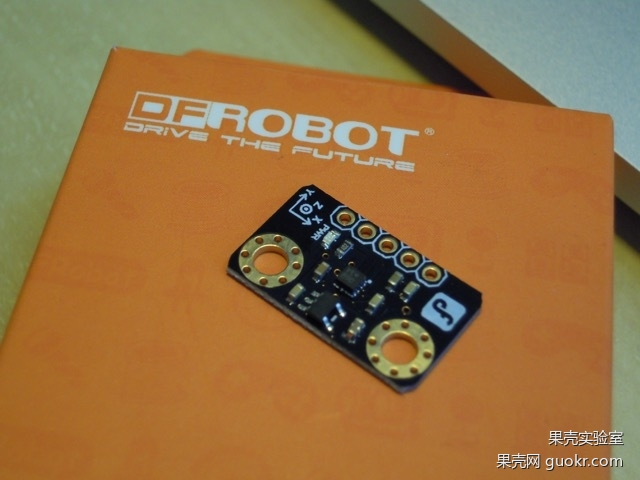【暴力快递评测报告】想知道哪家快递大叔更暴力？Arduino科学“跟踪”调查告诉你！DFRduino nanoBMA220加速度传感器

MiscroSD 模块

DS1307时间模块

3.7V 2000MA的锂电池与直流升压模块

```#include <Wire.h>
#include <math.h>
#include <SD.h>
#include <DS1307RTC.h>
#include <Time.h>
#include <Wire.h>

byte Version;
int8_t x_data;
int8_t y_data;
int8_t z_data;
byte range=0x03;
float divi=16;
float x,y,z;

File myFile;

void setup()
{
Serial.begin(9600);
Wire.begin();
Wire.beginTransmission(0x0A); // address of the accelerometer
// range settings
Wire.write(range); //can be set at"0x00""0x01""0x02""0x03", refer to Datashhet on wiki
// low pass filter
Wire.write(0x05); //can be set at"0x05""0x04"......"0x01""0x00", refer to Datashhet on wiki
Wire.endTransmission();

Serial.println("Initialization SD card...");
pinMode(10,OUTPUT);

if (!SD.begin(4)) {
Serial.println("initialization failed!");
return;
}
Serial.println("initialization done.");
}

void AccelerometerInit()// get accelerated
{
Wire.beginTransmission(0x0A); // address of the accelerometer
// reset the accelerometer
Wire.write(0x04); // Y data
Wire.endTransmission();
Wire.requestFrom(0x0A,1);    // request 6 bytes from slave device #2
while(Wire.available())    // slave may send less than requested
{
}
x_data=(int8_t)Version>>2;

Wire.beginTransmission(0x0A); // address of the accelerometer
// reset the accelerometer
Wire.write(0x06); // Y data
Wire.endTransmission();
Wire.requestFrom(0x0A,1);    // request 6 bytes from slave device #2
while(Wire.available())    // slave may send less than requested
{
}
y_data=(int8_t)Version>>2;

Wire.beginTransmission(0x0A); // address of the accelerometer
// reset the accelerometer
Wire.write(0x08); // Y data
Wire.endTransmission();
Wire.requestFrom(0x0A,1);    // request 6 bytes from slave device #2
while(Wire.available())    // slave may send less than requested
{
}
z_data=(int8_t)Version>>2;

x=(float)x_data/divi;
y=(float)y_data/divi;
z=(float)z_data/divi;
Serial.print("X=");
Serial.print(x);         // print the character
Serial.print("  ");
Serial.print("Y=");
Serial.print(y);         // print the character
Serial.print("  ");
Serial.print("Z=");           // print the character
Serial.print(z);
}

void loop()
{ double accelerated_speed;
switch(range)  //change the data dealing method based on the range u've set
{
case 0x00:divi=16;  break;
case 0x01:divi=8;  break;
case 0x02:divi=4;  break;
case 0x03:divi=2;  break;
default: Serial.println("range setting is Wrong,range:from 0to 3.Please check!");while(1);
}
AccelerometerInit();
accelerated_speed=sqrt(x*x+y*y+z*z);
Serial.print("accelerated_speed=");
Serial.println(accelerated_speed);
if(accelerated_speed>=1)
{
//get time
tmElements_t tm;
//write data
myFile = SD.open("test.txt", FILE_WRITE);
if (myFile) {
Serial.print("Writing to test.txt...");
//recoder time and data
myFile.print("accelerated_speed=");
myFile.print(accelerated_speed);
myFile.print("    ");
myFile.print("time=");
myFile.print(tm.Hour);//
myFile.print(':');
myFile.print(tm.Minute);//
myFile.print(':');
myFile.print(tm.Second);//
myFile.print(':');
myFile.print(", Date (D/M/Y) = ");
myFile.print(tm.Day);
myFile.write('/');
myFile.print(tm.Month);
myFile.write('/');
myFile.print(tmYear);
// close the file:
myFile.close();
Serial.println("done.");
} else {
// if the file didn't open, print an error:
Serial.println("error opening test.txt");
}
}
delay(100);
}
void print2digits(int number) {
if (number >= 0 && number < 10) {
Serial.write('0');
}
Serial.print(number);
}```

1#

2#

Anymous (专注于廉价迷你方便快捷的解决方案) | 2015-06-30 18:19

3#

@Anymous 嗯，geek，但是Arduino应该也很容易做出点hack的玩具

4#

sdc1992 | 2015-06-30 18:21

5#

@sdc1992 嗯，而且现在传感器对现实生活的信息感知能力非常强了，可以收集很多东西：P

6#

light (生于理想，死于欲望) | 2015-06-30 19:51

7#

zzzzy (我已然别无所求) | 2015-06-30 20:12

8#

Arduino 菜鸟玩家路过

9#

10#

11#

Knight (查水表。缴wb不杀) | 2015-07-01 09:47

12#

13#

@疯狗 @xsser 我想到的是当今快递行业对通信信号类设备还没有完善的检查措施，如果植入恶意的程序，被带到了快递总部车间，有没有完善的检测措施，能发生什么，还是可以想象的。

14#

@黑暗游侠 嗯，特殊的设备进入一个我们无法进入的区域，有点像电影

15#

4399gdww (人为刀俎我为鱼肉) | 2015-07-01 20:18

16#

17#

kow (我只是个酱油。。。) | 2015-07-02 11:40

18#